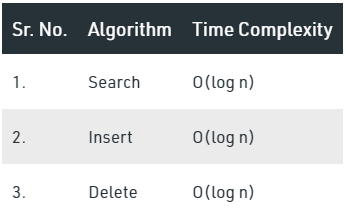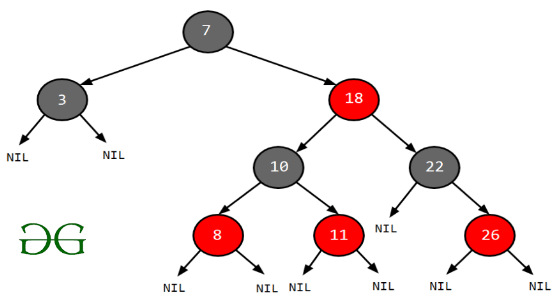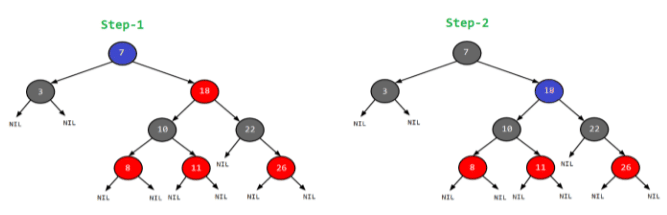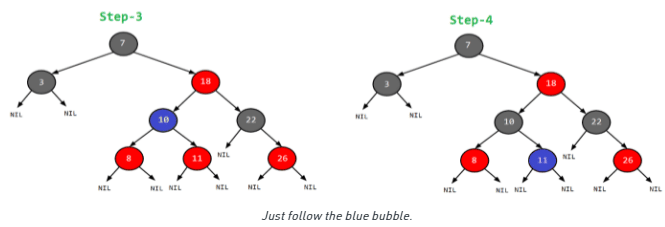Red-Black Trees

# Red-Black Trees Notes | Study Programming and Data Structures - Computer Science Engineering (CSE)

## Document Description: Red-Black Trees for Computer Science Engineering (CSE) 2022 is part of Programming and Data Structures preparation. The notes and questions for Red-Black Trees have been prepared according to the Computer Science Engineering (CSE) exam syllabus. Information about Red-Black Trees covers topics like Red-Black Tree | Set 1 (Introduction) and Red-Black Trees Example, for Computer Science Engineering (CSE) 2022 Exam. Find important definitions, questions, notes, meanings, examples, exercises and tests below for Red-Black Trees.

Introduction of Red-Black Trees in English is available as part of our Programming and Data Structures for Computer Science Engineering (CSE) & Red-Black Trees in Hindi for Programming and Data Structures course. Download more important topics related with notes, lectures and mock test series for Computer Science Engineering (CSE) Exam by signing up for free. Computer Science Engineering (CSE): Red-Black Trees Notes | Study Programming and Data Structures - Computer Science Engineering (CSE)
 1 Crore+ students have signed up on EduRev. Have you?

Red-Black Tree | Set 1 (Introduction)

Introduction:
A red-black tree is a kind of self-balancing binary search tree where each node has an extra bit, and that bit is often interpreted as the colour (red or black). These colours are used to ensure that the tree remains balanced during insertions and deletions. Although the balance of the tree is not perfect, it is good enough to reduce the searching time and maintain it around O(log n) time, where n is the total number of elements in the tree. This tree was invented in 1972 by Rudolf Bayer.

It must be noted that as each node requires only 1 bit of space to store the colour information, these types of trees show identical memory footprint to the classic (uncoloured) binary search tree.

Rules That Every Red-Black Tree Follows:
1. Every node has a colour either red or black.
2. The root of the tree is always black.
3. There are no two adjacent red nodes (A red node cannot have a red parent or red child).
4. Every path from a node (including root) to any of its descendants NULL nodes has the same number of black nodes.

Why Red-Black Trees?
Most of the BST operations (e.g., search, max, min, insert, delete.. etc) take O(h) time where h is the height of the BST. The cost of these operations may become O(n) for a skewed Binary tree. If we make sure that the height of the tree remains O(log n) after every insertion and deletion, then we can guarantee an upper bound of O(log n) for all these operations. The height of a Red-Black tree is always O(log n) where n is the number of nodes in the tree.“n” is the total number of elements in the red-black tree.

Comparison with AVL Tree:
The AVL trees are more balanced compared to Red-Black Trees, but they may cause more rotations during insertion and deletion. So if your application involves frequent insertions and deletions, then Red-Black trees should be preferred. And if the insertions and deletions are less frequent and search is a more frequent operation, then AVL tree should be preferred over Red-Black Tree.

How does a Red-Black Tree ensure balance?
A simple example to understand balancing is, a chain of 3 nodes is not possible in the Red-Black tree. We can try any combination of colours and see all of them violate Red-Black tree property.
A chain of 3 nodes is not possible in Red-Black Trees.
Following are NOT Red-Black Trees
30             30               30
/ \            /  \             /  \
20  NIL         20   NIL         20   NIL
/ \             / \              /  \
10  NIL          10  NIL          10  NIL
Violates          Violates        Violates
Property 4.      Property 4       Property 3

Following are different possible Red-Black Trees with above 3 keys
20                           20
/   \                        /   \
10     30                    10     30
/  \   /  \                 /  \    /  \
NIL  NIL NIL NIL             NIL  NIL NIL NIL

Interesting points about Red-Black Tree:
1. Black height of the red-black tree is the number of black nodes on a path from the root node to a leaf node. Leaf nodes are also counted as black nodes. So, a red-black tree of height h has black height >= h/2.
2. Height of a red-black tree with n nodes is h<= 2 log2(n + 1).
3. All leaves (NIL) are black.
4. The black depth of a node is defined as the number of black nodes from the root to that node i.e the number of black ancestors.
5. Every red-black tree is a special case of a binary tree.

Black Height of a Red-Black Tree :
Black height is the number of black nodes on a path from the root to a leaf. Leaf nodes are also counted black nodes. From the above properties 3 and 4, we can derive, a Red Black Tree of height h has black-height >= h/2.
Number of nodes from a node to its farthest descendant leaf is no more than twice as the number of nodes to the nearest descendant leaf.

Every Red Black Tree with n nodes has height <= 2Log2(n+1)

This can be proved using the following facts:
1. For a general Binary Tree, let k be the minimum number of nodes on all root to NULL paths, then n >= 2k – 1 (Ex. If k is 3, then n is at least 7). This expression can also be written as k <= Log2(n+1).
2. From property 4 of Red-Black trees and above claim, we can say in a Red-Black Tree with n nodes, there is a root to leaf path with at-most Log2(n+1) black nodes.
3. From property 3 of Red-Black trees, we can claim that the number of black nodes in a Red-Black tree is at least ⌊ n/2 ⌋ where n is the total number of nodes.

From the above points, we can conclude the fact that Red Black Tree with n nodes has height <= 2Log2(n+1)

Search Operation in Red-black Tree:
As every red-black tree is a special case of a binary tree so the searching algorithm of a red-black tree is similar to that of a binary tree.

Algorithm:
searchElement (tree, val)
Step 1:
If tree -> data = val OR tree = NULL
Return tree
Else
If val  data
Return searchElement (tree -> left, val)
Else
Return searchElement (tree -> right, val)
[ End of if ]
[ End of if ]
Step 2: END

Example: Searching 11 in the following red-black tree.Solution:
1. Start from the root.
2. Compare the inserting element with root, if less than root, then recurse for left, else recurse for right.
3. If the element to search is found anywhere, return true, else return false.In this post, we introduced Red-Black trees and discussed how balance is ensured. The hard part is to maintain balance when keys are added and removed. We have also seen how to search an element from the red-black tree. We will soon be discussing insertion and deletion operations in coming posts on the Red-Black tree.
Exercise:

1. Is it possible to have all black nodes in a Red-Black tree?
2. Draw a Red-Black Tree that is not an AVL tree structure-wise?

Insertion and Deletion
Red-Black Tree Insertion
Red-Black Tree Deletion

Applications:
1. Most of the self-balancing BST library functions like map and set in C++ (OR TreeSet and TreeMap in Java) use Red-Black Tree.
2. It is used to implement CPU Scheduling Linux. Completely Fair Scheduler uses it.
3. Besides they are used in the K-mean clustering algorithm for reducing time complexity.
4. Moreover, MySQL also uses the Red-Black tree for indexes on tables.

The document Red-Black Trees Notes | Study Programming and Data Structures - Computer Science Engineering (CSE) is a part of the Computer Science Engineering (CSE) Course Programming and Data Structures.
All you need of Computer Science Engineering (CSE) at this link: Computer Science Engineering (CSE)

## Programming and Data Structures

56 docs|16 tests
 Use Code STAYHOME200 and get INR 200 additional OFF

## Programming and Data Structures

56 docs|16 tests

Track your progress, build streaks, highlight & save important lessons and more!

,

,

,

,

,

,

,

,

,

,

,

,

,

,

,

,

,

,

,

,

,

;